JMSLTM Numerical Library 7.2.0
com.imsl.stat

## Class NormOneSample

• All Implemented Interfaces:
Serializable, Cloneable

```public class NormOneSample
extends Object
implements Serializable, Cloneable```
Computes statistics for mean and variance inferences using a sample from a normal population.

The statistics for mean and variance inferences are computed by using a sample from a normal population, including methods for the confidence intervals and tests for both mean and variance. The definitions of mean and variance are given below. The summation in each case is over the set of valid observations, based on the presence of missing values in the data.

Method `getMean`, returns value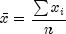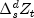Method `getStandardDeviation`, returns value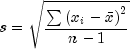The method `getTTestStat` returns the t statistic for the two-sided test concerning the population mean which is given by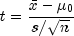where s and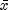are given above. This quantity has a T distribution with n - 1 degrees of freedom. The method `getTTestDF` returns the degree of freedom.

The method `getChiSquaredTestStat` returns the chi-squared statistic for the two-sided test concerning the population variance which is given by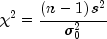where s is given above. This quantity has a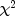distribution with n - 1 degrees of freedom. The method `getChiSquaredTestDF` returns the degrees of freedom.

Example, Serialized Form
• ### Constructor Summary

Constructors
Constructor and Description
`NormOneSample(double[] x)`
Constructor to compute statistics for mean and variance inferences using a sample from a normal population.
• ### Method Summary

Methods
Modifier and Type Method and Description
`double` `getChiSquaredTest()`
Returns the test statistic associated with the chi-squared test for variances.
`int` `getChiSquaredTestDF()`
Returns the degrees of freedom associated with the chi-squared test for variances.
`double` `getChiSquaredTestP()`
Returns the probability of a larger chi-squared associated with the chi-squared test for variances.
`double` `getLowerCIMean()`
Returns the lower confidence limit for the mean.
`double` `getLowerCIVariance()`
Returns the lower confidence limits for the variance.
`double` `getMean()`
Returns the mean of the sample.
`double` `getStdDev()`
Returns the standard deviation of the sample.
`double` `getTTest()`
Returns the test statistic associated with the t test.
`int` `getTTestDF()`
Returns the degrees of freedom associated with the t test for the mean.
`double` `getTTestP()`
Returns the probability associated with the t test of a larger t in absolute value.
`double` `getUpperCIMean()`
Returns the upper confidence limit for the mean.
`double` `getUpperCIVariance()`
Returns the upper confidence limits for the variance.
`void` `setChiSquaredTestNull(double chiSqrTestNull)`
Sets the null hypothesis value for the chi-squared test.
`void` `setConfidenceMean(double confidenceMean)`
Sets the confidence level (in percent) for a two-sided interval estimate of the mean.
`void` `setConfidenceVariance(double confidenceVariance)`
Sets the confidence level (in percent) for two-sided interval estimate of the variances.
`void` `setTTestNull(double meanHypothesis)`
Sets the Null hypothesis value for t test for the mean.
• ### Methods inherited from class java.lang.Object

`clone, equals, finalize, getClass, hashCode, notify, notifyAll, toString, wait, wait, wait`
• ### Constructor Detail

• #### NormOneSample

`public NormOneSample(double[] x)`
Constructor to compute statistics for mean and variance inferences using a sample from a normal population.
Parameters:
`x` - is a one-dimension `double` array containing the observations.
• ### Method Detail

• #### getChiSquaredTest

`public double getChiSquaredTest()`
Returns the test statistic associated with the chi-squared test for variances. The chi-squared test is a test of the hypothesis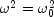where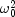is the null hypothesis value as described in `setChiSquaredTestNull`.
Returns:
a `double` containing the test statistic for the chi-squared test.
• #### getChiSquaredTestDF

`public int getChiSquaredTestDF()`
Returns the degrees of freedom associated with the chi-squared test for variances. The chi-squared test is a test of the hypothesiswhereis the null hypothesis value as described in `setChiSquaredTestNull`.
Returns:
an `int` the degrees of freedom for the chi-squared test.
• #### getChiSquaredTestP

`public double getChiSquaredTestP()`
Returns the probability of a larger chi-squared associated with the chi-squared test for variances. The chi-squared test is a test of the hypothesiswhereis the null hypothesis value as described in `setChiSquaredTestNull`.
Returns:
a `double` containing the probability of a larger chi-squared for the chi-squared test for variances.
• #### getLowerCIMean

`public double getLowerCIMean()`
Returns the lower confidence limit for the mean.
Returns:
a `double` containing the lower confidence limit for the mean.
• #### getLowerCIVariance

`public double getLowerCIVariance()`
Returns the lower confidence limits for the variance.
Returns:
a `double` containing the lower confidence limits for the variance.
• #### getMean

`public double getMean()`
Returns the mean of the sample.
Returns:
a `double` containing the mean.
• #### getStdDev

`public double getStdDev()`
Returns the standard deviation of the sample.
Returns:
a `double` containing the standard deviation of the sample.
• #### getTTest

`public double getTTest()`
Returns the test statistic associated with the t test. The t test is a test, against a two-sided alternative, of the null hypothesis value described in `setTTestNull`.
Returns:
a `double` containing the test statistic for the t test.
• #### getTTestDF

`public int getTTestDF()`
Returns the degrees of freedom associated with the t test for the mean. The t test is a test, against a two-sided alternative, of the null hypothesis value described in `setTTestNull`.
Returns:
an `int` containing the degrees of freedom for the t test.
• #### getTTestP

`public double getTTestP()`
Returns the probability associated with the t test of a larger t in absolute value. The t test is a test, against a two-sided alternative, of the null hypothesis value described in `setTTestNull`.
Returns:
a `double` containing the probability for the t test.
• #### getUpperCIMean

`public double getUpperCIMean()`
Returns the upper confidence limit for the mean.
Returns:
a `double` containing the upper confidence limit for the mean.
• #### getUpperCIVariance

`public double getUpperCIVariance()`
Returns the upper confidence limits for the variance.
Returns:
a `double` the upper confidence limits for the variance.
• #### setChiSquaredTestNull

`public void setChiSquaredTestNull(double chiSqrTestNull)`
Sets the null hypothesis value for the chi-squared test. The default is 1.0.
Parameters:
`chiSqrTestNull` - `double` containing the null hypothesis value for the chi-squared test.
• #### setConfidenceMean

`public void setConfidenceMean(double confidenceMean)`
Sets the confidence level (in percent) for a two-sided interval estimate of the mean.
Parameters:
`confidenceMean` - `double` containing the confidence level of the mean.

`confidenceMean` must be between 0.0 and 1.0 and is often 0.90, 0.95 or 0.99. For a one-sided confidence interval with confidence level c less than 50 percent, set `confidenceMean` =1.0- 2.0*(c/100).

This effectively gives the one-sided confidence interval for both c% and (100-c)%. For example, for a one-sided t-test with confidence level of 40, set ```confidenceMean ```=.2 . This means that 40% of the distribution is lower than confidence limit for the mean (`getLowerCIMean`) and 40% of the distribution is greater than the upper confidence limit for the mean (see getUpperCIMean). It also means that 60% of the distribution is greater than the lower confidence limit for the mean and 60% is lower than upper confidence limit for the mean. If the confidence mean is not specified, a 95-percent confidence interval is computed.

• #### setConfidenceVariance

`public void setConfidenceVariance(double confidenceVariance)`
Sets the confidence level (in percent) for two-sided interval estimate of the variances. Argument `confidenceVariance` must be between 0.0 and 1.0 and is often 0.90, 0.95 or 0.99. For a one-sided confidence interval with confidence level c (at least 50 percent), set `confidenceVariance`=1.0-2.0 * (1.0 - `c`). If the confidence mean is not specified, a 95-percent confidence interval is computed.
Parameters:
`confidenceVariance` - `double` containing the confidence level of the variance.
• #### setTTestNull

`public void setTTestNull(double meanHypothesis)`
Sets the Null hypothesis value for t test for the mean. `meanHypothesis`=0.0 by default.
Parameters:
`meanHypothesis` - `double` containing the hypothesis value.
JMSLTM Numerical Library 7.2.0